## Mahler-Lech Theorem

Let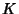be a Field of Characteristic 0 (e.g., the rationals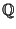) and letbe a Sequence of elements ofwhich satisfies a difference equation of the formwhere the Coefficients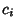are fixed elements of. Then, for any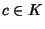, we have eitherfor only finitely many values of, orfor the values ofin some Arithmetic Progression.

The proof involves embedding certain fields inside the p-adic Number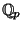for some Prime, and using properties of zeros of Power series over(Strassman's Theorem).